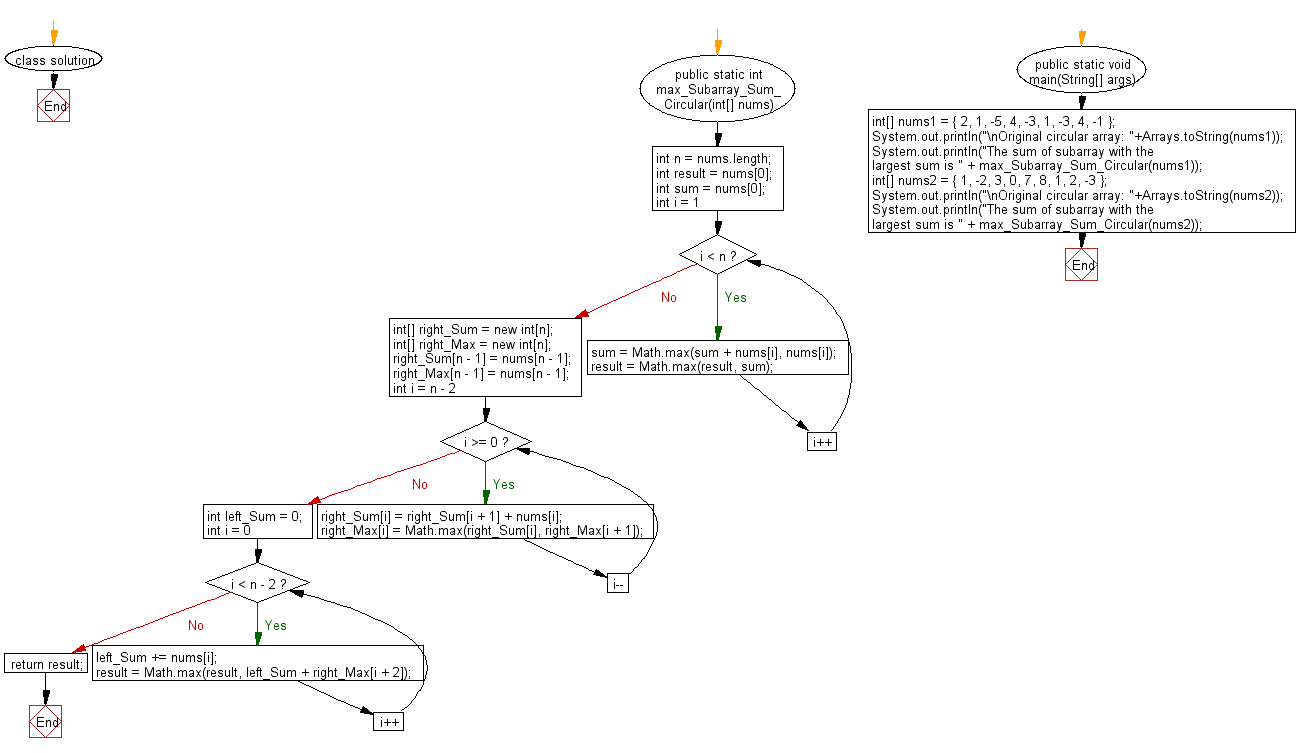﻿ Java: Subarray which has the largest sum in a circular array# Java Array Exercises: Find subarray which has the largest sum in a given circular array of integers

## Java Array: Exercise-67 with Solution

Write a Java program to find subarray which has the largest sum in a given circular array of integers.

Example:
Input :
nums1 = { 2, 1, -5, 4, -3, 1, -3, 4, -1 }
nums2 = { 1, -2, 3, 0, 7, 8, 1, 2, -3 }
Output:
The sum of subarray with the largest sum is 6
The sum of subarray with the largest sum is 21

Sample Solution:

Java Code:

``````import java.util.Arrays;
class solution {
public static int max_Subarray_Sum_Circular(int[] nums) {
int n = nums.length;
int result = nums;
int sum = nums;
for (int i = 1; i < n; i++) {
sum = Math.max(sum + nums[i], nums[i]);
result = Math.max(result, sum);
}

int[] right_Sum = new int[n];
int[] right_Max = new int[n];
right_Sum[n - 1] = nums[n - 1];
right_Max[n - 1] = nums[n - 1];
for (int i = n - 2; i >= 0; i--) {
right_Sum[i] = right_Sum[i + 1] + nums[i];
right_Max[i] = Math.max(right_Sum[i], right_Max[i + 1]);
}

int left_Sum = 0;
for (int i = 0; i < n - 2; i++) {
left_Sum += nums[i];
result = Math.max(result, left_Sum + right_Max[i + 2]);
}

return result;
}

public static void main(String[] args)
{
int[] nums1 = { 2, 1, -5, 4, -3, 1, -3, 4, -1 };
System.out.println("\nOriginal circular array: "+Arrays.toString(nums1));
System.out.println("The sum of subarray with the largest sum is " + max_Subarray_Sum_Circular(nums1));

int[] nums2 = { 1, -2, 3, 0, 7, 8, 1, 2, -3 };
System.out.println("\nOriginal circular array: "+Arrays.toString(nums2));
System.out.println("The sum of subarray with the largest sum is " + max_Subarray_Sum_Circular(nums2));
}
}
```
```

Sample Output:

```Original circular array: [2, 1, -5, 4, -3, 1, -3, 4, -1]
The sum of subarray with the largest sum is 6

Original circular array: [1, -2, 3, 0, 7, 8, 1, 2, -3]
The sum of subarray with the largest sum is 21
```

Flowchart:Java Code Editor:

Improve this sample solution and post your code through Disqus

What is the difficulty level of this exercise?

Test your Programming skills with w3resource's quiz.

﻿

## Java: Tips of the Day

countOccurrences

Counts the occurrences of a value in an array.

Use Arrays.stream().filter().count() to count total number of values that equals the specified value.

```public static long countOccurrences(int[] numbers, int value) {
return Arrays.stream(numbers)
.filter(number -> number == value)
.count();
}
```

Ref: https://bit.ly/3kCAgLb